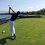# Another formula for squares

$\large{\text{Prove that}}$

$\large\sum_{n=1}^k (2n-1)=k^2$Note by Trevor Arashiro
6 years, 6 months ago

This discussion board is a place to discuss our Daily Challenges and the math and science related to those challenges. Explanations are more than just a solution — they should explain the steps and thinking strategies that you used to obtain the solution. Comments should further the discussion of math and science.

When posting on Brilliant:

• Use the emojis to react to an explanation, whether you're congratulating a job well done , or just really confused .
• Ask specific questions about the challenge or the steps in somebody's explanation. Well-posed questions can add a lot to the discussion, but posting "I don't understand!" doesn't help anyone.
• Try to contribute something new to the discussion, whether it is an extension, generalization or other idea related to the challenge.
• Stay on topic — we're all here to learn more about math and science, not to hear about your favorite get-rich-quick scheme or current world events.

MarkdownAppears as
*italics* or _italics_ italics
**bold** or __bold__ bold
- bulleted- list
• bulleted
• list
1. numbered2. list
1. numbered
2. list
Note: you must add a full line of space before and after lists for them to show up correctly
paragraph 1paragraph 2

paragraph 1

paragraph 2

[example link](https://brilliant.org)example link
> This is a quote
This is a quote
    # I indented these lines
# 4 spaces, and now they show
# up as a code block.

print "hello world"
# I indented these lines
# 4 spaces, and now they show
# up as a code block.

print "hello world"
MathAppears as
Remember to wrap math in $$ ... $$ or $ ... $ to ensure proper formatting.
2 \times 3 $2 \times 3$
2^{34} $2^{34}$
a_{i-1} $a_{i-1}$
\frac{2}{3} $\frac{2}{3}$
\sqrt{2} $\sqrt{2}$
\sum_{i=1}^3 $\sum_{i=1}^3$
\sin \theta $\sin \theta$
\boxed{123} $\boxed{123}$

## Comments

Sort by:

Top NewestSorry for bad drawing

- 6 years, 6 months ago

Log in to reply

Nice Megh !- 6 years, 5 months ago

Log in to reply

Impressive!

- 6 years, 6 months ago

Log in to reply

Thanks dear friend

- 6 years, 6 months ago

Log in to reply

How do you come up with such beautiful thinking!? I bow before you! Lovely representation!

- 6 years, 6 months ago

Log in to reply

Sorry for late response , thought about this long back

- 6 years, 5 months ago

Log in to reply

EXACTLY, This is the pattern that I saw which prompted me to make this note.

- 6 years, 6 months ago

Log in to reply

Let $S_{O} = \displaystyle\sum_{n=1}^{k} (2n - 1)$ and $S_{E} = \displaystyle\sum_{n=1}^{k} (2n)$.

Then $S_{E} - S_{O} = k$ and $S_{O} + S_{E} = \displaystyle\sum_{n=1}^{2k} n = \dfrac{2k(2k + 1)}{2} = 2k^{2} + k$.

Therefore $(S_{O} + S_{E}) - (S_{E} - S_{O}) = (2k^{2} + k) - k \Longrightarrow 2S_{O} = 2k^{2} \Longrightarrow S_{O} = k^{2}$.

- 6 years, 6 months ago

Log in to reply

Nice one! Did you know this from before of did you just derive this in two minutes?

- 6 years, 6 months ago

Log in to reply

No, I just saw the pieces, put them together and out came the desired result. :)

- 6 years, 6 months ago

Log in to reply

I will use proof by induction to show that if the formula is true for p(k) then it is true for p(k+1) and hence it is true for all natural numbers. Let s(k) denote the sum for values up to k. Base step: s(1) = 1, s(2) = 1+3 = 4 ... so the formula is true so far, as no counter-examples have been found. Inductive step: s(k+1) = $k^{2}$ + 2(k+1) -1 = $k^{2}$ +2k +1 = $(k+1)^{2}$, as required. Q.E.D.

- 6 years, 6 months ago

Log in to reply

starting with n=1, (21 - 1) + (22-1) + (23-1) +. . . . . . . . . (2k -1) 21 -1+ 22 - 1 +23 -1 +.............. 2k - 1 22 + 22 + 23 ....2k - k1 2(1 + 2+ 3+ ......k) - k using AP sum formula;{1 + 2+ 3+...k= k/2(21 +k-1) = k/2(1+k) keeping values, =2*k/2(1+k) - k = k^2 Q.E.D

- 6 years, 6 months ago

Log in to reply

sorry a small doubt from childhood , seen in many books , what does Q.E.D means?

- 6 years, 6 months ago

Log in to reply

It's quad era demonstrandum which means "which was to be demonstrated"

- 6 years, 6 months ago

Log in to reply

The "alternative" explanation is "Quite Easily Done". :)

Staff - 6 years, 5 months ago

Log in to reply

that was a sarcastic remark I guess.

- 6 years, 5 months ago

Log in to reply

It's a running joke in the Math community. See for example, Wikipedia Q.E.D. Modern humorous usage

Q.E.D. is sometimes jokingly claimed to abbreviate "quite easily done", or "Quit. Enough done."

Note: I generally avoid making sarcastic remarks over the internet, unless the context is very easily deduced. his is because sacarsm doesn't transfer well.

Staff - 6 years, 5 months ago

Log in to reply

You could also do it from $r^2-(r-1)^2=2r-1$ and them sum both sides from $r=1$ to $r=k$. The LHS just telescopes and gives you $k^2$ and the RHS becomes the LHS of the given q :-)

- 6 years, 5 months ago

Log in to reply

I agree that it would provide a nice alternative as $\displaystyle \sum_{i=1}^n$ $r^{2}$ - $(r-1)^{2}$ = $\frac{n(n+1)(2n+1)}{6}$ - $\frac{n(n-1)(2n-1)}{6}$ = $\frac{n}{6}$ [(2$n^{2}$ +3${n}$ +1) - ((2$n^{2}$ -3${n}$ +1)] = $n^{2}$, which is less wordy :)

- 6 years, 5 months ago

Log in to reply

×

Problem Loading...

Note Loading...

Set Loading...